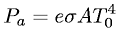Equations > Physics > Thermodynamics > Radiation absorbed by an object

### Radiation absorbed by an objectLatex Code:

MathML Code:

 ${P}_{a}=e\sigma {\mathrm{AT}}_{0}^{4}$

MathType 5.0: